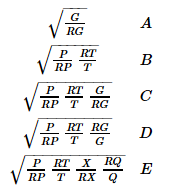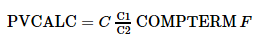# C195 – AP09-600 – Flow Rate Calculation (Compensation Method)

## Description

This calculation is used to calculate flow rate using a choice of algorithms commonly used by distributed control systems (DCS).

Honeywell – Advanced Process Manager Control Functions and Algorithms – AP09-600

Kelton calculation reference C195

FLOCALC calculation reference F052

KIMS calculation reference K195

## Options

### Primary Variable

• Mass
• Volume
• Energy

Select option based on desired flow parameter.

### Input Method

• Linear Value
• % Linear
• % Square root

Select option based on whether the uncompensated flowrate is a linear or square rooted term.

### Calculation Method

• A: Liquids
• B: Gasses and Vapours
• C: Gasses and Vapours with Specific Gravity
• D: Volumetric Flow of Gasses and Vapours
• E: Steam

Select option based on properties of the measured fluid.

## Calculation

### Flow compensation term

The flow compensation term is calculated by:Where A, B, C, D, E = Refer to the calculation method for which the formula is employed G = Specific gravity RG = Design Specific gravity P = Pressure RP = Design Pressure T = Temperature RT = Design Temperature X = Steam Compressibility RX = Design Steam Compressibility Q = Steam Quality Factor RQ = Design Steam Quality Factor

Note: If input method is linear square root is not necessary.

### Compensated flow rate

The flow rate is calculated by:Where C = Scale Factor C1 = 1st correction constant C2 = 2nd correction constant F = Uncompensated flow input COMPTERM = Flow compensation term

Back